Next: Inclusion of Multiple Scattering Up: Track Fitting in r- Previous: Track Fitting in r-

## Pivot Transformation in Vacuum

We will show below that in the high momentum limit the effects of multiple scattering can be implemented in a continuous manner. For this purpose, it turns out very useful to set up machinary to move the pivot, taking into account the effects of multiple scattering.

We first consider the pivot transformation without multiple scattering. The track parameter vector (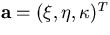)transforms under an infinitesimal change of pivot: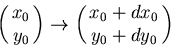as follows: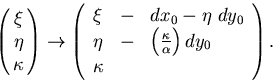This can be cast into a vector equation: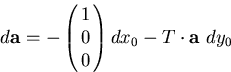(42)
where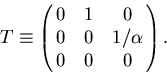(43)
From now on, we keep dx0 = 0, which reduces the pivot transformation to a single parameter group and the above equation to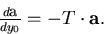(44)
Under this condition,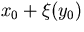represents the x coordinate of the track at y = y0. In terms of the generator T, the transformation matrix for the error matrix is given by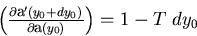(45)
which implies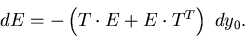(46)Next: Inclusion of Multiple Scattering Up: Track Fitting in r- Previous: Track Fitting in r-
Keisuke Fujii
12/4/1998